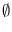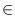Next: Bounded Node Priority Queues Up: Graphs and Related Data Previous: Node Partitions ( node_partition   Contents   Index

# Node Priority Queues ( node_pq )

Definition

An instance Q of the parameterized data type node_pq<P> is a partial function from the nodes of a graph G to a linearly ordered type P of priorities. The priority of a node is sometimes called the information of the node. For every graph G only one node_pq<P> may be used and every node of G may be contained in the queue at most once (cf. section Priority Queues for general priority queues).

#include < LEDA/graph/node_pq.h >

Creation

 node_pq

Q(const graph_t& G) creates an instance Q of type node_pq

for the nodes of graph G with dom(Q) =.

Operations

 void Q.insert(node v, const P& x) adds the node v with priority x to Q. Precondition vdom(Q). const P& Q.prio(node v) returns the priority of node v. Precondition vdom(Q). bool Q.member(node v) returns true if v in Q, false otherwise. void Q.decrease_p(node v, const P& x) makes x the new priority of node v. Precondition x < = Q.prio(v). node Q.find_min() returns a node with minimal priority (nil if Q is empty). void Q.del(node v) removes the node v from Q. node Q.del_min() removes a node with minimal priority from Q and returns it (nil if Q is empty). node Q.del_min(P& x) as above, in addition the priority of the removed node is assigned to x. void Q.clear() makes Q the empty node priority queue. int Q.size() returns | dom(Q)|. int Q.empty() returns positive integer if Q is the empty node priority queue, 0 otherwise. const P& Q.inf(node v) returns the priority of node v.

Implementation

Node priority queues are implemented by binary heaps and node arrays. Operations insert, del_node, del_min, decrease_p take time O(log m), find_min and empty take time O(1) and clear takes time O(m), where m is the size of Q. The space requirement is O(n), where n is the number of nodes of G.Next: Bounded Node Priority Queues Up: Graphs and Related Data Previous: Node Partitions ( node_partition   Contents   Index